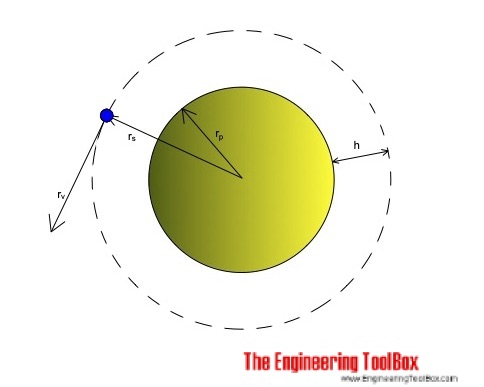Engineering ToolBox - Resources, Tools and Basic Information for Engineering and Design of Technical Applications!

# Satellites in Orbit

## Orbital and escape velocity for geostationary satellites.Orbital velocity of a satellite is at maximum at sea level and decreases with height.

Orbital velocity can be calculated as

vs = (g rp2 / rs)1/2                                (1)

where

vs = orbital velocity (m/s)

g = acceleration due to gravity (m/s2) (9.81 m/s2)

rp = radius planet (m) (earth: 6.37 106 m)

rs = radius satellite orbit (m)

Maximum velocity at sea level where radius planet equals radius orbit can be expressed as

vs_max = (g rp)1/2                                (1b)

Escape velocity where the satellite will leave its orbit and escape the planet gravity can be calculated as

vs_escape = (2 g rp)1/2                                (2)

Orbit periodic time can be expressed as

ts = 2 π (rs3 / (g rp2))1/2                         (3)

where

ts = orbit time (s)

Height of orbit can be calculated as

hs = rp ((g ts2 / (4 π2 rp))1/3 - 1)                     (4)

where

hs = height of orbit (m)

### Example - Earth bound Satellites

Maximum velocity at sea level:

vs_max = ((9.81 m/s2) (6.37 106 m))1/2

= 7905 m/s

= 7.9 km/h

Escape velocity at sea level:

vs_escape = (2 (9.81 m/s2) (6.37 106 m))1/2

= 11179 m/s

= 11.2 km/h

Height of the synchronous orbit for a geostationary satellite can be calculated by using (4) for an orbit period of 24 hours or 86400 s:

hs = (6.37 106 m) (((9.81 m/s2) (86400 s)2 / (4 π2 (6.37 106 m)))1/3 - 1)

= 35968 km

## Related Topics

• ### Miscellaneous

Engineering related topics like Beaufort Wind Scale, CE-marking, drawing standards and more.

## Related Documents

• ### Latitude and Longitude Converter

Convert between Degrees, Minutes and Seconds and Decimal Units (or vice versa).
• ### Projectile Range

Calculate the range of a projectile - a motion in two dimensions.
• ### Solar System Constants

Properties and data for the Sun, the Earth and the Moon.

## Engineering ToolBox - SketchUp Extension - Online 3D modeling!

Add standard and customized parametric components - like flange beams, lumbers, piping, stairs and more - to your Sketchup model with the Engineering ToolBox - SketchUp Extension - enabled for use with older versions of the amazing SketchUp Make and the newer "up to date" SketchUp Pro . Add the Engineering ToolBox extension to your SketchUp Make/Pro from the Extension Warehouse !

We don't collect information from our users. More about

## Citation

• The Engineering ToolBox (2019). Satellites in Orbit. [online] Available at: https://www.engineeringtoolbox.com/satellites-orbital-velocity-height-escape-d_2173.html [Accessed Day Month Year].

Modify the access date according your visit.

9.29.12